Courses

Test: Probability- 1

20 Questions MCQ Test Quantitative Aptitude for GMAT | Test: Probability- 1

Description
Attempt Test: Probability- 1 | 20 questions in 40 minutes | Mock test for GMAT preparation | Free important questions MCQ to study Quantitative Aptitude for GMAT for GMAT Exam | Download free PDF with solutions
QUESTION: 1

What is the probability that a person born between the years 1990 and 2010, inclusive, was born in a leap year?

Solution:

Step I: Define Event

The event here is that the person was born in a leap year between 1990 and 2010, inclusive. We know that a leap year is a year that is divisible by 4.

Step II: Find n, the number of ways in which all outcomes can occur

Since the boundary years 1990 and 2010 are included as well, the total number of years to be considered is 2010 – 1990 + 1 = 21.

(Note: Suppose the years given were 1990-1992 inclusive, then how many years would that be? 3 years: 1990, 1991 and 1992. But 1992-1990 is equal to only 2. Therefore, when the boundary years are included, the correct formula to count the number of years will be: Final Year – Initial Year + 1)

The person may be born in any of these years.

Thus, n = 21C1 = 21

Step III: Find x, the number of ways in which the event can occur

To calculate x, we need to find the leap years between 1990 and 2010, inclusive.

We know that a number is divisible by 4 if its last two digits are divisible by 4. Using this rule, we can determine that the leap years will be:

1992,  1996, 2000, 2004 and 2008

Thus, there are 5 leap years. The person being born in any of these 5 years will be a favorable outcome.

Therefore, x = 5

Step IV: Calculate probability

P = x/n = 5/21

QUESTION: 2

From a set of consecutive numbers from 1 to 20 (both inclusive) a number is selected.  What is the probability that the selected number is divisible by 5?

Solution:

Step I: Define the Event

We are given a set of 20 numbers. A single number is selected from this set. The event will be the selection of a number that is divisible by 5

Step II: Find n, the number of ways in which all outcomes can occur

As we know there are 20 numbers in the set. Any of these numbers may be selected.

Thus, n = 20C1 = 20

Step III: Find x, the number of ways in which the event can occur

To find x, we should list the numbers between 1 and 20 that are divisible by 5. These numbers are:

5, 10, 15 and 20.

Thus, there are 4 numbers between 1 and 20 that are divisible by 5.

The event will occur if any of these 4 numbers is selected.

Thus, x =  4C1 = 4

Step IV: Probability = x/n

P = x/n = 4/20 = 1/5

So, the probability of the selected number being divisible by 5 is 1/5.

QUESTION: 3

An unbiased die is rolled once. What is the probability of getting a prime number?

Solution:

Step I: Define the Event

We are given that a die is rolled and we have to find the probability of getting a prime number on the top face of the die. So, the event in this case is the show of a prime number on the top face of the die.

Step II: Find n, the number of ways in which all outcomes can occur

Now, the total number of possible outcomes is 6 i.e. the show of 1, 2, 3, 4, 5, or 6 on the face of the die.

So, n = 6

Step III: Find x, the number of ways in which the event can occur

Next, we are going to find the number of ways in which the event can occur. These number of ways are also called favorable outcomes.

The favorable outcomes here are the show of the prime numbered faces. So, basically we have to find the number of prime numbers in the range 1-6.

There are total three prime numbers 2, 3, and 5 in the given range.

There are 3 favorable outcomes.

So, x = 3

Step IV: Probability = x/n

In this step, we are going to calculate the probability by plugging the values of x and n in the Probability formula.

P = x/n = 3/6 = ½ = 0.5

So, the probability of getting a prime number on rolling an unbiased die is 0.5

QUESTION: 4

A bag contains 7 different black and 5 different white balls. If one ball is selected at random find the probability that the ball drawn is white.

Solution:

Step I: Define the Event

We are given that a bag contains 7 black and 5 white balls and we have to find the probability of selecting a white ball if only one ball is selected.

Thus, the event in this case is selecting one white ball.

Step II: Find n, the number of ways in which all outcomes can occur

As we know, there are 7 + 5= 12 balls in the bag. Now, the number of ways in which one ball can be selected from a bag containing 12 balls is 12C1 = 12

Thus, n = 12

Step III: Find x, the number of ways in which the event can occur

Next, we are going to find the number of ways in which the event can occur.

The favorable outcome here is selecting a white ball. Now, the number of ways in which a white ball can be selected out of 5 white balls = 5C1 = 5

There are 5 favorable outcomes.

Step IV: Probability = x/n

P = x/n = 5/12

So, the probability of selecting a white ball from a bag containing 7 black and 5 white balls is 5/12.

QUESTION: 5

Two dice, one colored white and the other colored yellow, are rolled simultaneously. What is the probability of getting a sum greater than or equal to 10?

Solution:

Step I: Define the Event

We are given that two dice are rolled together and we have to find out the probability of getting a sum greater than or equal to 10.

Thus, the event in this case is getting the sum of the top faces of the two dice greater than or equal to 10.

Step II: Find n, the number of ways in which all outcomes can occur

As we know two dice are rolled together and we have to find out the sum of their top faces. So, the total number of possible outcomes are as follows:

= (Total number of outcomes on the white die) * (Total number of the outcomes on the yellow die)

= 6 * 6 = 36

Thus, n = 36

Step III: Find x, the number of ways in which the event can occur

Next, we are going to find the number of ways in which the event can occur.

The favorable outcomes here are the show of the faces whose total is greater than or equal to 10. This condition is satisfied when the sum of the two numbers is 10, 11, or 12. Note that, the sum cannot be more than 12 because the highest number on the face of a die can be 6 only.

So, the number of ways in which the sum can be

10 = (5, 5), (6, 4), (4, 6)  => Total number of ways = 3

11 = (5, 6), (6, 5)  => Total number of ways = 2

12 = (6, 6)  => Total number of ways = 1

There are total 6 possible outcome that give us the sum as greater than or equal to 10.

x = 6

Step IV: Probability = x/n

P = x/n = 6/36 = 1/6

So, the probability of getting the sum greater than or equal to 10 on rolling two unbiased different dice is 1/6.

(Note: Since the two dice are different, getting 6 on the white dice and 4 on the yellow dice is a different case than getting 4 on the white dice and 6 on the yellow dice. Had the two dice been exactly similar to one another, (4,6) would have been counted as only 1 case and not as 2 separate cases as we have done here. In the probability questions that involve multiple dices, you should assume that the dices are different unless explicitly told otherwise)

QUESTION: 6

There are 10 houses in the neighborhood, each equally likely to foreclose. One of these houses is adjacent to Sanchez' house.  A recent study has shown that if an adjacent house forecloses, the value of a house drops by \$10,000. If it is predicted that one house will foreclose each week, what is the probability that the value of Sanchez' house will drop by \$10,000 this week?

Solution:

Step I: Define the Event

Here, we have to find the probability of the event that the value of Sanchez’s house drops by \$10,000. This drop in value will come if a house adjacent to Sanchez forecloses.

Thus, the event in this case is: the house adjacent to Sanchez forecloses

Step II: Find n, the number of ways in which all outcomes can occur

The number of ways in which any one of the 10 houses can foreclose, n = 10.

Step III: Find x, the number of ways in which the event can occur

The event happens if the house adjacent to Sanchez’s forecloses. This can happen only in one way since there is only one house adjacent to Sancehz’ house.

Thus, x = 1

Step IV: Probability = x/n

P (Event) = 1/10 = 0.1

QUESTION: 7

A box contains 26 blocks, each listing a unique English alphabet.  If 3 blocks are picked at a time, what is the probability that all of them are vowels?

Solution:

If three alphabets are selected at once from all the alphabets, we have to find the probability that all the three alphabets are vowels. This is equivalent to: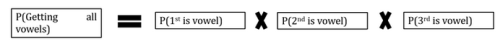Step B: Find probabilities of individual events

Event A: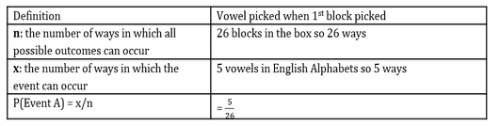Now, as we know that picking things together is equivalent to picking things one by one without replacement. So, when one vowel is picked out of the 26 alphabets, we are left with a total of 25 alphabets including 4 vowels.

Event B: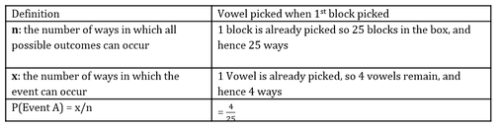Now, since two vowels are picked out of the 26 alphabets, we are left with a total of 24 alphabets including 3 vowels.

Event C: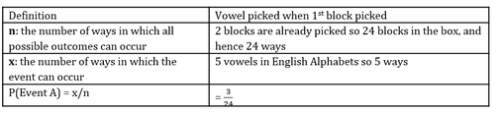Step C: Plug all P(Events) in the main equation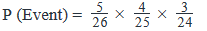QUESTION: 8

4 coins are tossed.  What is the probability of getting neither all heads nor all tails?

Solution:

Step 1: Probability Event Equation

Here, since the number of favorable outcomes (you get some heads and some tails but not all heads and all tails) is far more than the number of unfavorable outcomes (there is only one way in which you can get either all heads or all tails), we will use the non-event equation to find the Probability of the event:

P(Event) = 1 -P(Non-Event)

Step 2: Define Event

Event = Neither all heads nor all tails

=> Non-Event = All heads or all tails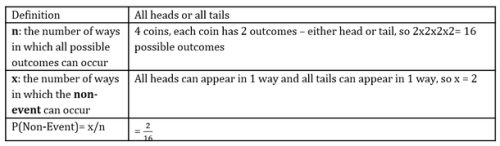Step 3: Plug all P(Events) in the main equation:

P(Event) = 1 -2/16 = 1-1/8 = 7/8

Looking at the answer choices, we see that Option C is the correct choice.

QUESTION: 9

A dance group consists of 6 men and 7 women.  There are 3 married couples in the group.  If 2 people have to be selected – 1 man and 1 woman, what is the probability that one of the couples will be selected?

Solution:

Step 1: Define the Event

Consider 6 men in the group to be M1 M2 M3 M4 M5 M6

Consider 7 women in the group to be W1 W2 W3 W4 W5 W6 W7

The group has 3 married couples.

(Consider (M1, W1) (M2, W2) and (M3, W3) to be the married couples.)

1 man out of the 6 men in the group &

1 woman out of the 7 women in the group are to be selected.

The Event is that one of the 3 married couples gets selected.

Step 2: Find n, the number of ways in which all outcomes can occur

•        Total number of ways in which 1 man can be selected out of 6 men           = 6C1 = 6
•        Total number of ways in which 1 woman can be selected out of 7 women  = 7C1 = 7

Thus,

•        Total number of possible outcomes, n = 6 x 7 = 42

(For example, selections like: (M1, W7) (M2, W5) (M1, W1) ……........................... etc. are all possible)

Note here that we use a multiplication sign in the above equation because we need to select both a man AND a woman.

Step 3: Find x, the number of ways in which the event can occur

The Event is the selection of a married couple.

In how many ways you can select a married couple out of 3 married couples??

•        No: of ways in which 1 married couple can be selected out of 3 married couples
•        3C1 = 3

Thus,

•        x = 3

Step 4: Probability = x/n

Therefore,

•        Probability (a married couple is selected) = x/n = 3/42 = 1/14

In other words,

Out of the 42 possible selections ((M1, W7) (M2, W5) (M1, W1) …................…. etc.),

there are 3 selections ((M1, W1) (M2, W2) and (M3, W3)) who will be married couples.

QUESTION: 10

Two dice are rolled simultaneously. What is the probability of getting a sum greater than or equal to 10?

Solution:

Step I: Define the Event

We are given that two dice are rolled together and we have to find out the probability of getting a sum greater than or equal to 10.

Thus, the event in this case is getting the sum of the top faces of the two dice greater than or equal to 10.

Step II: Find n, the number of ways in which all outcomes can occur

The total number of possible outcomes are as follows:

= (Total number of outcomes on the first die) * (Total number of the outcomes on the second die)

= 6 * 6 = 36

Thus, n = 36

Step III: Find x, the number of ways in which the event can occur

The favourable outcomes here are the show of the faces whose total is greater than or equal to 10. This condition is satisfied when the sum of the two numbers is 10, 11, or 12. Note that, the sum cannot be more than 12 because the highest number on the face of a die can be 6 only.

So, the number of ways in which the sum can be

10 = (5, 5), (6, 4), (4, 6)  => Total number of ways = 3

11 = (5, 6), (6, 5)  => Total number of ways = 2

12 = (6, 6)        => Total number of ways = 1

There are total 6 possible outcome that give us the sum as greater than or equal to 10.

There are 6 favourable outcomes.

Thus, x = 6

Step IV: Probability = x/n

P = x/n = 6/36 = 1/6

So, the probability of getting the sum greater than or equal to 10 on rolling two unbiased dice is 1/6.

QUESTION: 11

A team of 4 people is to be formed from a group of 4 girls and 4 boys. Find the probability that the team consists of at least one girl.

Solution:

Step I: Define non-event

We are given that there are 8 people in a group: 4 girls and 4 boys. A team of 4 people is to be formed such that there is at least one girl in the team.

So, the events in this case are:

Event 1: One girl in the team

Event 2: Two girls in the team

Event 3: Three girls in the team

Event 4: Four girls in the team

Thus, there are 4 events that we’ll have to consider to solve this question. This is going to be quite a tedious calculation!

On the other hand, let’s see how many non-events are there?

Only 1: No girl in the team.

Since the number of non-events is far less than the number of events, it’s easier to solve this question using the non-event method.

P (At least one girl in the team) = 1 – P (No girls in the team)

Step II: Find n, the number of ways in which all outcomes can occur

As we know, there are 4 + 4 = 8 people in the team. So, the number of ways in which a team of 4 people can be selected from 8 persons is 8C4 = 70

Thus, n = 70

Step III: Find x, the number of ways in which the non-event can occur

Next, we are going to find the number of ways in which the non-event can occur. The non-event will occur when no girl is included in the team.

The number of ways to select a team of 4 people so that no girl is included in the team = Selecting a team of 4 people from the 4 boys only

= 4C4 = 1

Thus, x = 1

Step IV: Calculate probability of non-event

In this step, we are going to calculate the probability by plugging in the values.

P (No girl is selected)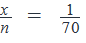Step V: Probability (Event) = 1-Probability (Non-event)

Now, let’s put the value of P (Non-event) in the probability event equation:

P (At least one girl in the team) = 1 – P (No girls in the team)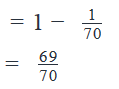QUESTION: 12

Two letters from the word FARTHING are selected at random. What is the probability that at least one of the selected letters is a consonant?

Solution:

Step I: Define Non-event

We are given that two letters are selected from the word FARTHING.

The word FARTHING contains 2 Vowels (A, I) and 6 consonants (F, R, T, H, N, G)

We need to find the probability that at least one of the selected letters is a consonant.

So, the events in this case are:

Event 1: One of the two selected letters is a consonant and the other is a vowel

Event 2: Both the selected letters are consonants.

On the other hand, there is only one non-event:

Non-event: None of the selected letters is a consonant.

So, it’ll be easier to solve this question using the Non-Event method.

The Probability Event Equation will therefore be:

P(at least one of the selected letters is a consonant) = 1 – P(none of the selected letters is a consonant)

Step II: Find n, the number of ways in which all outcomes can occur

Now, the word FARTHING consists of 8 different letters. Of these, 2 are selected.

Thus, the total number of outcomes, n = 8C2 = 28

Step III: Find x, the number of ways in which the Non-event can occur

The non-event is that none of the selected letters is a consonant.

This means, the selected letters can only be vowels.

We have 2 vowels available: A and I.

There is only one way in which 2 vowels can be selected from 2 vowels (2C2 =1)

So, x = 1

Step IV: Calculate probability for Non-event

P(none of the selected letters is a consonant)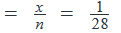Step V: Probability (Event) = 1-Probability (Non-event)

Now, let’s put the value of P (Non-event) in the probability event equation:

P(at least one of the selected letters is a consonant) = 1 – P(none of the selected letters is a consonant)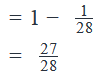QUESTION: 13

A Science test and an English test were administered to a class of 30 students. Half of the students passed in Science, 60% of the students passed in English and 10% failed in both. If a student is selected at random from the class, what is the probability that the student has passed in at least one of the two tests?

Solution:

Step I: Define Non-event

1 student is selected at random out of a class of 30 students. The event in this question is that the selected student has passed in at least one of the two tests.

Since a majority of the students have passed at least one of the two sets (it is given that only 10% of the students have failed in both tests), the number of ways in which the event can occur will be far more than the number of ways in which the non-event can occur.

So, this question will be solved more quickly through the non-event method.

The non-event in this case will be that the selected student has failed in both the tests.

So, the probability event equation is:

P(Student has passed at least 1 test) = 1 – P(Student has failed both tests)

Step II: Find n, the number of ways in which all outcomes can occur

Now, the total number of ways in which 1 student can be selected out of 30 students

= 30C1 = 30

So, n = 30

Step III: Find x, the number of ways in which the Non-event can occur

The non-event is the selection of a student who has failed both tests.

From the question statement, we know that

Number of students who failed both tests = 10% of all students

= 10% of 30 = 3

Thus, the non-event occur if one of out of these 3 students is selected.

Number of ways in which this can happen = 3C1 = 3

So, x = 3

Step IV: Calculate probability for Non-event

P(Student has failed both tests)

Step V: Probability (Event) = 1-Probability (Non-event)

Now, let’s put the value of P (Non-event) in the probability event equation:

P(Student has passed at least 1 test) = 1 – P(Student has failed both tests)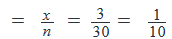Step V: Probability (Event) = 1-Probability (Non-event)

Now, let’s put the value of P (Non-event) in the probability event equation:

P(Student has passed at least 1 test) = 1 – P(Student has failed both tests)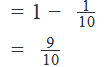QUESTION: 14

A bag contains 8 balls, of which 4 are white and the remaining are red. Three balls are selected from the bag at random without replacement. Find the probability that at least one of the three selected balls is red.

Solution:

Step I: Define Non-event

We are given that from a bag containing 4 red and 4 white balls, three balls are selected at random without replacement. We have to find the probability that at least one red ball is selected.

Now, following are the events in this case:

Event 1: One red ball is selected

Event 2: Two red balls are selected

Event 3: Three red balls are selected

However, there is only one non-event i.e. no red ball is selected. So, it will be easier to solve this question using the non-event approach.

So, we can write the probability event equation as:

P (At least one red ball is selected) = 1 – P (No red ball is selected)

Step II: Find n, the number of ways in which all outcomes can occur

Now, the total number of ways in which 3 balls can be selected from 8 balls is:

= 8C3 = 56

So, n = 8C3 = 56

Step III: Find x, the number of ways in which the Non-event can occur

Next, we are going to find the number of ways in which the non-event can occur.

As mentioned above, the non-event is that no red ball is selected among the three balls. This implies that the three selected balls are all white.

Now, the total number of white balls is 4.

So, we have to find the number of ways in which 3 white balls can be selected from 4 white balls.

Thus, x = 4C3 = 4

Step IV: Calculate probability for Non-event

P(No red ball is selected)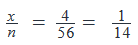Step V: Probability (Event) = 1-Probability (Non-event)

P (At least one red ball is selected) = 1 – P (No red ball is selected)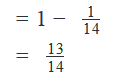QUESTION: 15

A card is drawn from a pack of cards, in which all the cards are equally likely to be drawn. Find the probability that the card drawn is not a face card.

Solution:

Step I: Define Non-event

We are given that a card is drawn from a pack of cards. We have to find the probability that the card drawn is not a face card.

(Note: The term face card is used to denote a card that depicts a person. These cards are: King, Queen and Jack)

The event here is that the drawn card is NOT a face card.

The non-event is the opposite of the event.

So, the non-event in this case is to draw a face card from the pack.

The Probability Event Equation will be:

P(face card is not drawn) = 1 – P (face card is drawn)

Step II: Find n, the number of ways in which all outcomes can occur

Now, the total number of ways in which a card can be drawn from a pack of 52 cards:

= 52C1 = 52

Thus, n = 52

Step III: Find x, the number of ways in which the Non-event can occur

To find x, we need to determine the total number of face cards in a pack of cards.

A pack of card has 4 suits: Hearts, Spades, Diamonds and Clubs

Each suit has 3 face cards: King, Queen and Jack

So, total number of face cards = 3 * 4 = 12

Now, the number of ways in which a face card can be selected out of the 12 face cards = 12C1 = 12

Thus, x = 12

Step IV: Calculate probability for Non-event

Here, we are going to calculate the probability of the non-event by plugging in the values of x and n.

P(face card is drawn)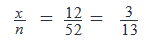Step V: Probability (Event) = 1-Probability (Non-event)

Now, let’s put the value of P (Non-event) in the probability event equation:

P(face card is not drawn) = 1 – P (face card is drawn)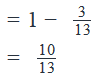QUESTION: 16

There are 12 pens and 5 pencils in box A . An object is picked at random from box A and moved to box B , in which there are already 4 pens and 5 pencils. If one object is then to be picked from box B , what is the probability that a pen will be picked?

Solution:

A. Write Probability Event Equation

We are given that there are two boxes A and B. Both the boxes contain some pens and pencils.

First, an object from box A is selected randomly and put into box B.

Then, one object is selected from box B. We have to find the probability that this selected object is a pen.

So, there are two possible scenarios:

Event 1: A pen is picked from box A and a pen is picked from box B

Event 2: A pencil is picked from box A and a pen is picked from box B

Since the objective of selecting a pen can be completed by accomplishing either Event 1 OR Event 2, the Probability Event Equation will be:

P(Selecting a pen from B) = P(Event 1) + P(Event 2)

B. Determine Probabilities of Individual Events

Event 1

Step I: Define Event

We have already defined this event to be: A pen is picked from box A and a pen is picked from box B

We are given that box A contains 12 pens and 5 pencils and box B contains 4 pens and 5 pencils.

Event 1 consists of the following two sub-events:

1. Picking a pen from box A and putting it in box B,
2. Picking a pen from box B (By now, box B contains 4+1 = 5 pens)

Step II: Find n1, the number of ways in which all outcomes can occur

As we know there are total 12 + 5 =17 objects in box A. Now, the total number of ways in which an object can be picked from this box is = 17C1 = 17

The total number of ways in which an object can be picked from box B = 10C1 =10

Since we have to perform both the above actions, we will use a multiplication sign to determine the total number of outcomes.

So, the total possible outcomes are = 17*10 =170

Thus, n1 =170

Step III: Find x1, the number of ways in which the event can occur

The favorable outcome from box A is the one in which a pen is selected. Now, the number of ways in which a pen can be selected from 12 pens = 12C1 = 12

The favorable outcomes from box B is if a pen is selected. Now, the number of ways in which a pen can be selected from 5 pens = 5C1 = 5

Since we have to perform both the above actions, we will use a multiplication sign to determine the total number of favorable outcomes.

So, the total number of favorable outcomes are 12*5 = 60

Thus, x1 = 60

Step IV: Calculate probability

P (Event 1)  =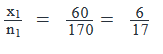Event 2

Step I: Define Event

We are given that box A contains 12 pens and 5 pencils and box B contains 4 pens and 5 pencils. Per Event 2, a pencil is picked from box A and put in box B. So, box B now contains 4 pens and 6 pencils.

Now, Event 2 consists of the following two sub-events:

1. Picking a pencil from box A and putting it in box B,
2. Picking a pen from box B.

Step II: Find n2, the number of ways in which all outcomes can occur

As we know there are total 12 + 5 =17 objects in box A. Now, the total number of ways in which an object can be picked from this box is = 17C1 = 17

The total number of ways in which an object can be picked from box B = 10C1 =10

Since we have to perform both the above actions, we will use a multiplication sign to determine the total number of outcomes.

So, the total possible outcomes are = 17*10 =170

Thus, n2 =170

Step III: Find x2, the number of ways in which the event can occur

The favorable outcome from box A is the one in which a pencil is selected. Now, the number of ways in which a pencil can be selected from 5 pencils = 5C1 = 5

The favorable outcomes from box B is if a pen is selected. Now, the number of ways in which a pen can be selected from 4 pens = 4C1 = 4

Since we have to perform both the above actions, we will use a multiplication sign to determine the total number of favorable outcomes.

So, the total number of favorable outcomes are 5*4 = 20

Thus, x2 = 20

Step IV: Calculate probability

P (Event 2) =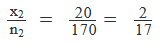C.    Plug the values in the Probability Event Equation

P(Selecting a pen from B) = P(Event 1) + P(Event 2)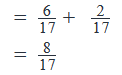QUESTION: 17

A and B are members of a group of 11 people. A team of 6 members is to be chosen from this group. What is the probability that A and B will either both be in the team or will both be out of the team?

Solution:

A. Write Probability Event Equation

We are given that 6 persons are to be chosen from a group of 11 people. This group of 11 people includes A and B. We have to find the probability that either both A and B will be in the team or neither of them will be in the team.

So, the events in this case are:

Event 1: Both A and B are in the team

Event 2: None of A and B is in the team

The objective of the question can be completed either by including A and B in the team OR by excluding both of them from the team. Therefore, we will put an addition sign between the probabilities of the two events in the Probability Event Equation:

P(Selectingateamof6peopleundergivenconstraint)=P(Event1)+P(Event2)

B. Determine Probabilities of Individual Events

Event 1

Step I: Define Event

We are given that there are 11 people in a group, and 6 people are chosen randomly from the group to form a team.

Event 1 is that both A and B are chosen in the team.

Step II: Find n1, the number of ways in which all outcomes can occur

As mentioned above, the team of 6 is to be chosen from a group of 11 people. Now, the total number of ways in which the team can be chosen is =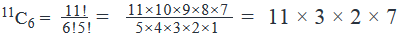Thus, n1 = 11×3×2×7

Step III: Find x1, the number of ways in which the event can occur

The favorable outcome for this event is that both A and B are included in the 6-member team. So, we need to perform two tasks here:

1. Select A and B in the team
2. Select the remaining 4 members of the team

Now, we have to select A and B in the team from a given pool of two people: A and B. So, total number of ways = 2C2 = 1

After A and B have been taken in the team, 9 people remain in the group. Of these 9 people, we need to select 4 for the team.

The number of ways in which 4 members can be selected from a group of 9 = 9C4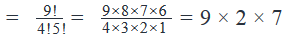Since we have to complete both the tasks to make a team of 6 members, we will put a multiplication sign between the number of ways of doing the two tasks.

Thus, x1 =1×9×2×7=9×2×7

Step IV: Calculate probability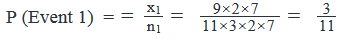So, the probability of event 1 is 3/11

Event 2

Step I: Define Event

We are given that there are 11 people in a group, and 6 people are chosen randomly from the group to form a team.

Event 2 is that both A and B are NOT chosen in the team.

Step II: Find n2, the number of ways in which all outcomes can occur

As mentioned above, the team of 6 is to be chosen from a group of 11 people. Now, the total number of ways in which the team can be chosen is =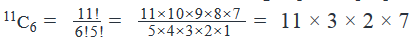Thus, n2 = 11×3×2×7

Step III: Find x2, the number of ways in which the event can occur

The favorable outcome for this event is that both A and B are NOT included in the team. So, we need to choose a team of 6 members from a group of 9 people.

(Note that, since A and B are not to be included in the team, we have only 9 people left from which the team of 6 is to be selected.)

Thus, the number of ways in which 6 members can be selected from a group of 9 = 9C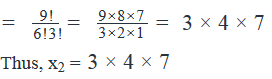Step IV: Calculate probability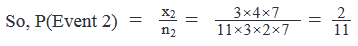C. Plug the values in the Probability Event Equation

P(Selectingateamof6peopleundergivenconstraint)=P(Event1)+P(Event2)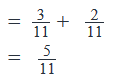QUESTION: 18

Four persons enter a lift on the ground floor of an 8-floor building(excluding the ground floor). Each person is equally likely to leave the lift on any floor except the ground floor. What is the probability of all the persons leaving the lift on different floors?

Solution:

A. Write Probability Event Equation

We are given that 4 persons enter a lift on the ground floor of an 8-floor building. Every person in the lift is equally likely to leave the lift on any floor except the ground floor. Let’s say the persons are P1, P2, P3 and P4.

We need to find the probability of all the 4 persons leaving the lift on different floors.

So, the events in this case are:

Event 1: P1 leaves the lift at a floor (other than the ground floor).

Total 8 options (Any floor from 1-8)

Event 2: P2 leaves the lift at any floor (other than the one taken by P1)

Total 7 options (Any floor from 1-8 except P1's floor)

Event 3: P3 leaves the lift at any floor (other than the ones taken by P1, P2)

Total 6 options (Any floor from 1-8 except P1's floor and P2's floor)

Event 4: P4 leaves the lift at any floor (other than the ones taken by P1, P2, P3)

Total 5 options (Any floor from 1-8 except P1's floor, P2's floor, and P3's floor)

Since the objective of the question can be completed only if all the four persons leave the lift, all the four events need to happen for the objective to be accomplished. So, the sign of multiplication will be used here in the Probability Event Equation:

P(all4leavingatdifferentfloors)=P(Event1)×P(Event2)×P(Event3)×P(Event4)

B. Determine Probabilities of Individual Events

Event 1

• The favorable outcome for P1 is to leave the lift on any floor.
• Now, the number of ways in which P1 can select a floor from 8 floors = 8C1=8
• As we know there are total 8 floors on which P1 can leave the lift.
• Now, the total number of ways in which P1 can leave the lift is = 8C1 = 8
• Probability = 8/8 = 1

Event 2

• The favorable outcome for P2 is to leave the lift on any floor (other than the one taken by P1).
• Now, the number of ways in which P2 can select a floor from the remaining 7 floors = 7C1 = 7
• The total number of ways in which P2 can leave the lift is = 8C1 = 8
• Probability = 7/8

Event 3

• The favorable outcome for P3 is to leave the lift on any floor (other than the ones taken by P1 and P2).
• Now, the number of ways in which P3 can select a floor from the remaining 6 floors = 6C1=6
• The total number of ways in which P3 can leave the lift is = 8C1 = 8
• Probability = 6/8 = 3/4

Event 4

• The favorable outcome for P4 is to leave the lift on any floor (other than the ones taken by P1, P2 and P3).
• Now, the number of ways in which P4 can select a floor from the remaining 5 floors = 5C1= 5
• The total number of ways in which P4 can leave the lift is = 8C1 = 8
• Probability = 5/8

C. Plug the values in the Probability Event Equation

P(all4leavingatdifferentfloors)=P(Event1)×P(Event2)×P(Event3)×P(Event4)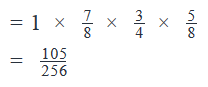QUESTION: 19

Three squares on a chessboard are chosen at random. The probability that 2 of them are of one color and the remaining one is of another color is:

Solution:

A. Write Probability Event Equation

We are given that 3 squares on a chessboard are chosen at random. We have to find the probability that 2 of these squares are of one color and the remaining one is of another color.

A chessboard has 8 rows and 8 columns. So, there are total 8*8 = 64 squares on the chessboard. Out of these 64 squares, 32 are black and 32 are white.

So, the events in this case are:

Event 1: Selecting two black squares and one white square

Event 2: Selecting one black squares and two white square

Since the objective of the question can be completed by accomplishing either Event 1 OR Event 2, we will use an addition sign in the Probability Event Equation:

P(Selecting3squaresonachessboardundergivenconstraint)=P(Event1)+P(Event2)

B. Determine Probabilities of Individual Events

Event 1

Step I: Define Event

There are 64 squares on the chessboard, of which 32 are black and 32 are white.

Event 1 is to select 2 black squares and 1 white square.

Step II: Find n1, the number of ways in which all outcomes can occur

The total possible outcomes in this case is equal to the number of ways to select any 3 squares from the chessboard. So, the number of ways in which 3 squares can be selected from 64 squares

= 64C3

Thus, n1 = 64C3

Step III: Find x1, the number of ways in which the event can occur

To accomplish Event 1, we have to do the following tasks:

1. Select 2 black squares
2. Select one white square

The number of ways to select 2 black squares from 32 black squares = 32C2

The number of ways to select 1 white square from 32 white squares = 32C1

Since both these tasks need to be completed to accomplish the objective of Event 1, we will use a multiplication sign between the number of ways of doing the two events:

Thus, x1 = 32C2 * 32C1

Step IV: Calculate probability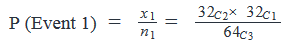NOTE: Order doesn't matter here - Hence, it is a Selection Question.

For Example:

Better Method (Combination): We will just select 2 black and 1 white square.

OR

Lengthy Method (Permutation): We consider all the following 3 cases:

1) We select first white and then 2 blacks OR

2) We select first 2 blacks and then white.

3) we select first a black, then white and then a black.

Event 2

Step I: Define Event

There are 64 squares on the chessboard, of which 32 are black and 32 are white.

Event 2 is to select 1 black square and 2 white squares.

Similar to Event 1, Order doesn't matter here.

Step II: Find n2, the number of ways in which all outcomes can occur

The total possible outcomes in this case is equal to the number of ways to select any 3 squares from the chessboard. So, the number of ways in which 3 squares can be selected from 64 squares

= 64C3

Thus, n2 = 64C3

Step III: Find x2, the number of ways in which the event can occur

To accomplish Event 2, we have to do the following tasks:

1. Select 1 black square
2. Select 2 white squares

The number of ways to select 1 black square from 32 black squares = 32C1

The number of ways to select 2 white squares from 32 white squares = 32C2

Since both these tasks need to be completed to accomplish the objective of Event 2, we will use a multiplication sign between the number of ways of doing the two events: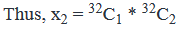Step IV: Calculate probability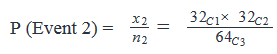C. Plug the values in the Probability Event Equation

P(Selecting 3 squares on a chess board undergiven constraint) =P (Event1) +P (Event2)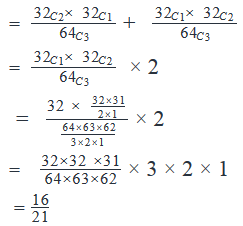QUESTION: 20

Alex participates in a shooting competition and makes n attempts to shoot a target. Is the probability that he will shoot the target in all the n attempts that he makes, greater than 50%?

1. Alex has a 80% chance of shooting the target in every attempt that he makes.
2. He makes 7 attempts in total.
Solution:

This is a DS question on the concept of probability. So, we will apply the A-B-C approach that we have learnt for Probability questions within the 5-step framework that we universally apply for all DS questions.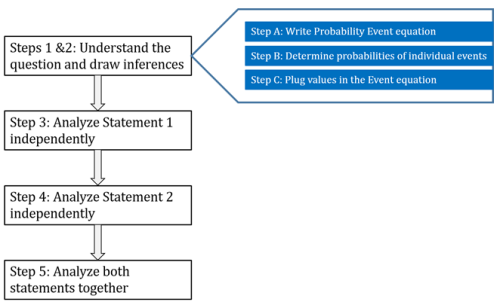Steps 1&2: Understand the question and draw inferences

We know that Alex makes n attempts to shoot the target.

We need to determine the probability that he shoots the target in each of the n attempts.

Step A: Write Probability Event Equation

In order to do so, we should list all the events.

Event 1: Alex shoots the target in 1st attempt

Event 2: Alex shoots the target in 2nd attempt, and so on. . .

Event n: Alex shoots the target in nth attempt

Now, we should determine whether ‘AND’ or ‘OR’ applies.

The question statement asks about the probability of Alex shooting the target in everyattempt. So, he needs to shoot the target in the 1st attempt AND in the 2nd attempt and so on. . .

So, the Probability Event Equation will be:

P(Alexshootingthetargetineveryattempt)=P(Event1)×P(Event2)×...×P(Eventn)

Step B: Determine Probabilities of individual events

From the question statement, we do not know:

i)         What is the probability of his shooting the target in every attempt

ii)       Whether the probability of his shooting the target is equal for every attempt or different

So, let us assume that:

The probability of his shooting the target the first attempt = P1

The probability of his shooting the target in the second attempt = P2  . . .

The probability of his shooting the target in the nth attempt = Pn

Step C: Plug values of Individual Event Probabilities in the Event Equation

Thus, the Event Equation becomes:

P(Alexshootingthetargetineveryattempt)=P1×P2×...×Pn

.......Equation 1

The question asks us whether P(Alex shooting the target in every attempt) is greater than 0.50

In order to answer this question, we need to know the values of P1, P2  . . . Pn

Step 3: Analyze Statement 1 independently

Alex has 80% chance of hitting the target in every attempt that he makes

From this statement, we get:

P1 = P2 = . .  . Pn  = 0.80

So, Equation 1 becomes:

P(Alex shooting the target in each attempt) = 0.8× 0.8× 0.8...n times

P(Alexshootingthetargetineachattempt)=(0.8)n

Since we do not know the value of n, we will not be able to determine if

P(Alex shooting the target in each attempt ) >0.5

Thus, Statement 1 alone is not sufficient to answer the question.

Step 4: Analyze Statement 2 independently

He makes 7 attempts in total

From this statement, we get n = 7

Thus, from Equation 1, we get:

P(Alex shooting the target in each attempt) =P1×P2×...×P7

However, this statement gives us no clue about the values of P1, P2  . . . P7

Thus, Statement 2 alone is clearly not sufficient to answer the question.

Step 5: Analyze both statements together (if needed)

From Statement 1,

P(Alex shooting the target in each attempt) =(0.8)n

From Statement 2,

n = 7

By combining both statements, we get:

P(Alex shooting the target in each attempt) =(0.8)7

From this equation, we will be able to determine the exact numerical value of the probability that Alex shoots the target in every attempt. Therefore, we will also be able to determine if this value is greater than 0.50 or not.

Thus, both statements together are sufficient to answer the question.Use Code STAYHOME200 and get INR 200 additional OFF Use Coupon Code

Track your progress, build streaks, highlight & save important lessons and more!

Similar ContentRelated tests